# PSAT Math : How to find a solution to a compound fraction

## Example Questions

### Example Question #1 : General Fractions

Simplify: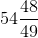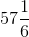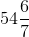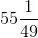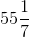Explanation: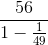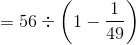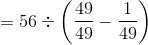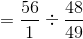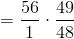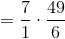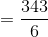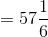### Example Question #2 : General Fractions

Simplify: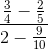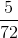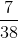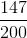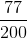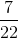Explanation: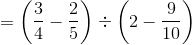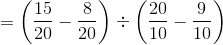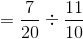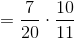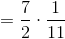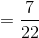### Example Question #3 : General Fractions

Simplify: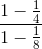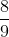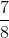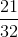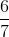Explanation: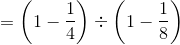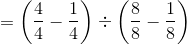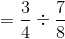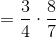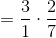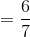### Example Question #4 : General Fractions

Simplify: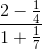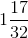None of the other responses gives the correct answer.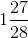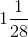Explanation: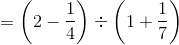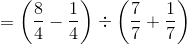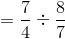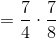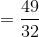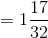### Example Question #5 : General Fractions

Simplify: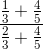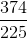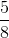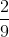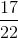Explanation: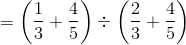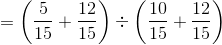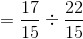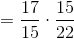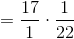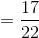### Example Question #141 : Fractions

Simplify: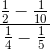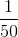The correct answer is not given among the other responses.The correct answer is not given among the other responses.

Explanation: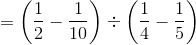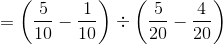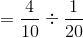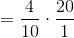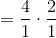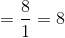This answer is not among the given choices.